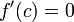# First derivative test is conclusive for locally analytic function

This article describes a situation, or broad range of situations, where a particular test or criterion is conclusive, i.e., it works as intended to help us determine what we would like to determine.
The test is first derivative test. See more conclusive cases for first derivative test | inconclusive cases for first derivative test

## Statement

Suppose$f$ is a function,$c$ is a point in the interior of the domain of$f$, and$f$ is analytic about$c$, i.e., there is a power series centered at$c$ that converges to$f$ on an open interval containing$c$. In particular, this means that$f$ is infinitely differentiable at$c$. The assumption of$c$ being a critical point also forces$f'(c) = 0$.

In this case, the first derivative test is conclusive for$f$.

## Proof

The proof follows directly by applying Fact (1) to the derivative$f'$ and combining with Fact (2).